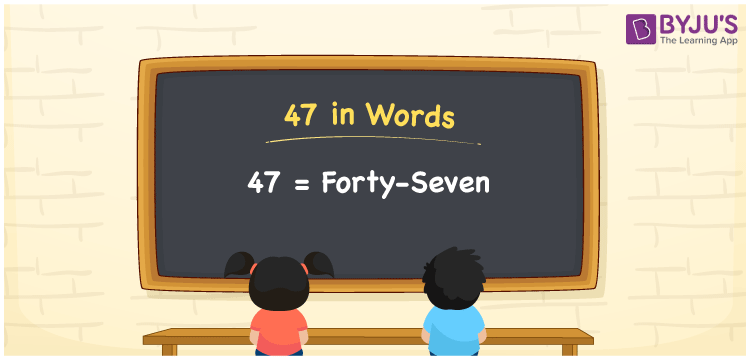# 47 in Words

47 in words can be written as Forty-seven. If you buy a dish at the restaurant for Rs. 47, then you can say that “I bought a dish for Forty-seven Rupees”. In this article, students will get a clear cut knowledge of how to count numbers and its uses in our daily lives. To write numbers in words, the faculty have made use of the English alphabet. Therefore, the number 47 can be read as “Forty-seven” in words.

 47 in words Forty-seven Forty-seven in Numbers 47

## 47 in English Words## How to Write 47 in Words?

The concept of a place value chart for 47 in words is explained in simple language. Two digits are present in the number 47. The place value chart indicating the place value of two digits is mentioned here.

 Tens Ones 4 7

The expanded form of 47 is provided below:

4 × Ten + 7 × One

= 4 × 10 + 7 × 1

= 40 + 7

= 47

= Forty-seven

Therefore, 47 in words is written as Forty-seven.

47 is a natural number that precedes 48 and succeeds 46.

47 in words – Forty-seven

Is 47 an odd number? – Yes

Is 47 an even number? – No

Is 47 a perfect square number? – No

Is 47 a perfect cube number? – No

Is 47 a prime number? – Yes

Is 47 a composite number? – No

## Frequently Asked Questions on 47 in Words

Q1

### How do you write the number 47 in words?

47 in words is Forty-seven.
Q2

### Find the value of 50 minus 3.

The value of 50 minus 3 is 47. Therefore, 47 in words is Forty-seven.
Q3

### Is 47 a perfect square?

No, 47 is not a perfect square as it cannot be expressed as the product of two integers which are equal.